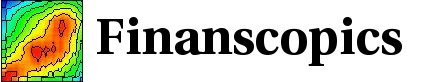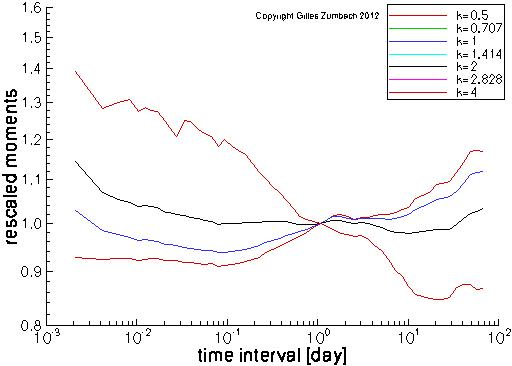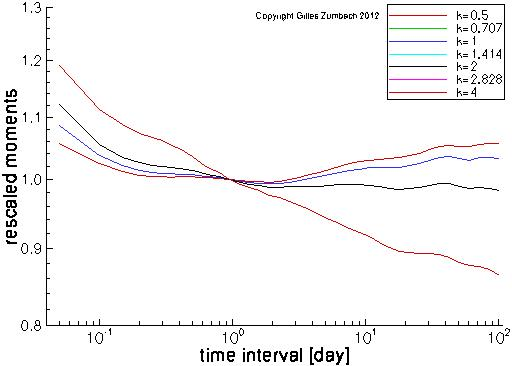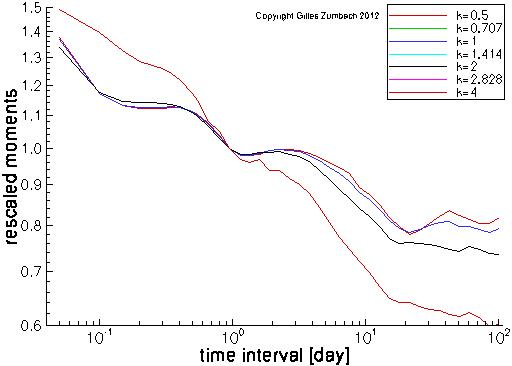Base information Indexes

# Scaling for the moments: description

## Normalized moments of the return

The horizontal axis gives the time horizons of the returns, the vertical axis gives the normalized moments.For a given exponent k, the moment E[|r[dt]|k]1/k of the returns at various time interval dt. The exponent k takes the values 0.5, 1, 2 and 4 (the caption is incorrect!). Each curve is multiplied by a constant such that the moment at dt = 1 day is equal to 1. The graph scales are log-log, in order to search for power laws.

Remember that the definition of the returns already includes a factor (1y/dt)1/2, and therefore the usual random walk scaling is already discounted. Thanks to this convenient normalization, these moment scaling curves capture the deviations from a random walk.

In roughly 2 decades from 0.1 to 10 day, the moments are nearly linear (in log-log scales), indicating a power law behavior (multifractal). The scaling for k < 2 (k > 2) show a positive (negative) slope, while the moment for k=2 is nearly flat (black line).

Notice also the different behaviors at very short and very long time intervals.

## Normalized moments of the volatility

The horizontal axis gives the time horizons of the volatility, the vertical axis gives the normalized moments.The moments computations (see above) for the volatility. Notice that the moments are not centered, namely the mean is not subtracted (contrary say to a standard deviation).

## Normalized moments of the volatility increment

The horizontal axis gives the time horizons of the volatility increment, the vertical axis gives the normalized moments.The moments computations (see above) for the volatility increment.